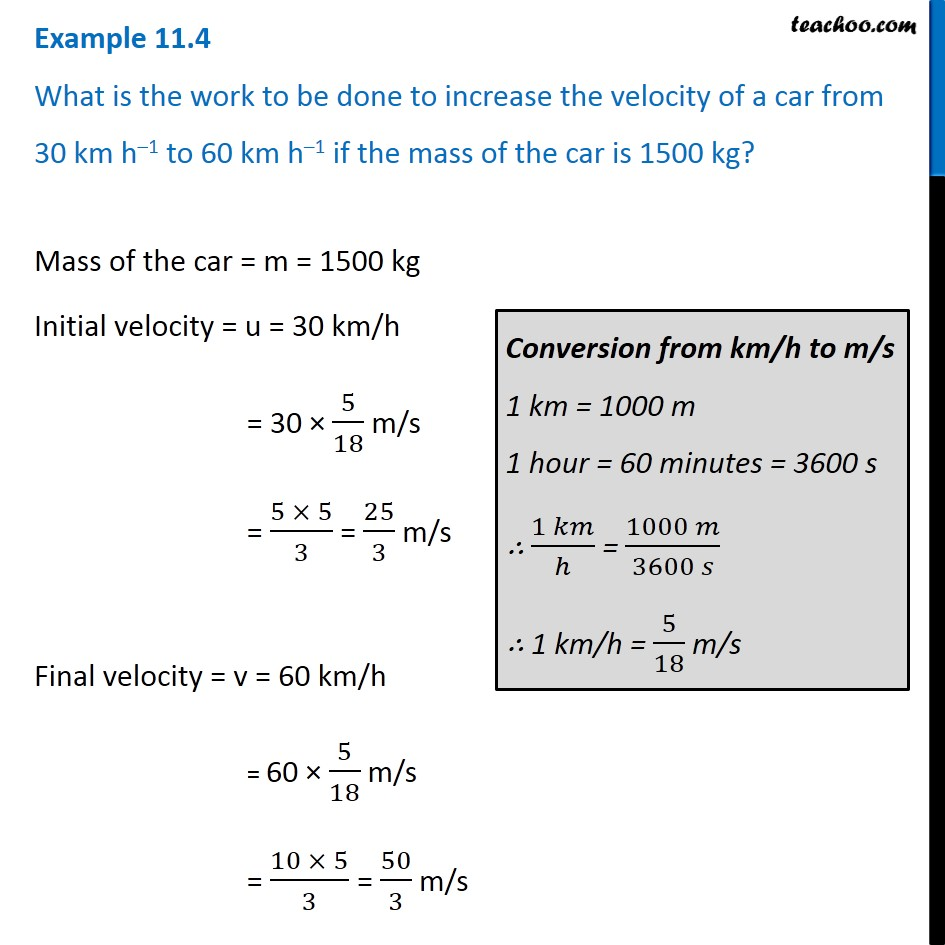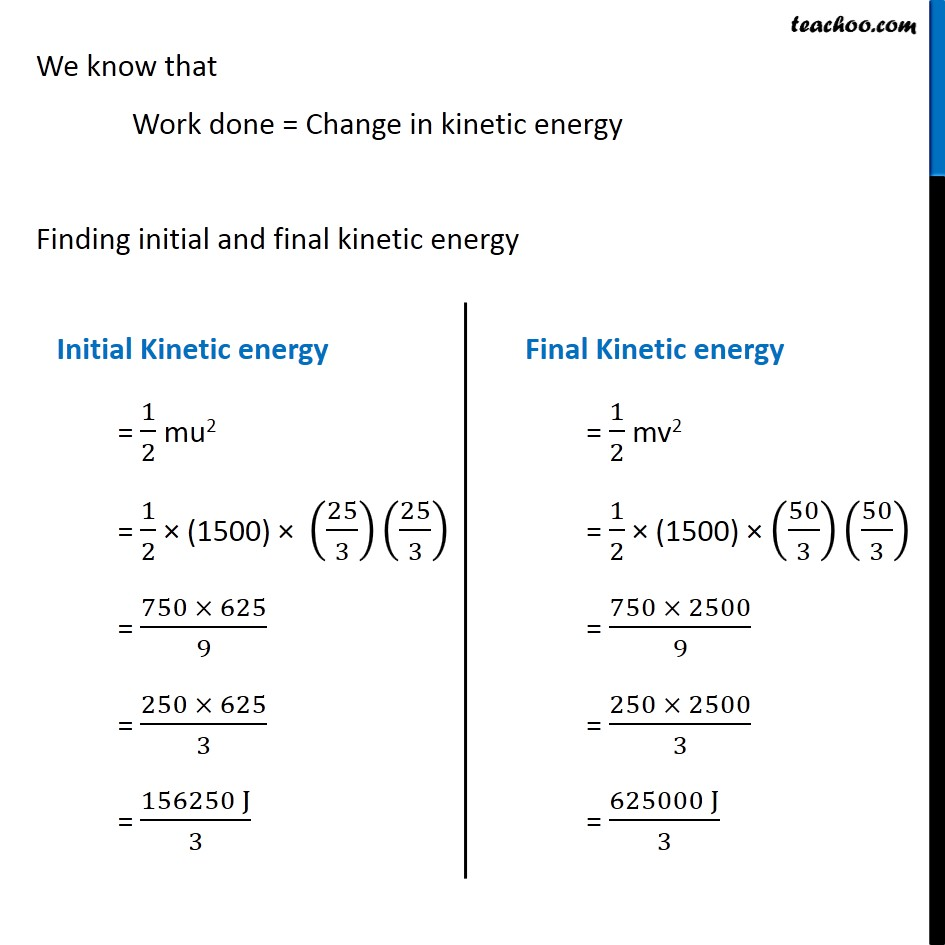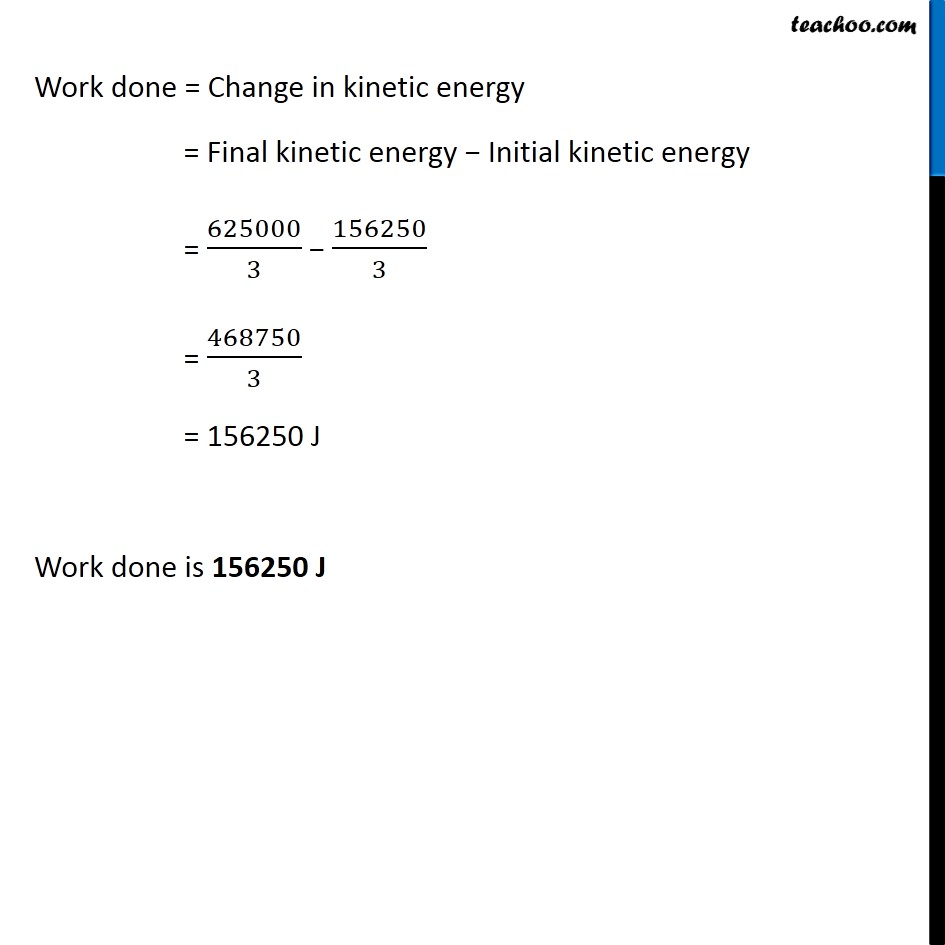Examples from NCERT Book

Class 9
Chapter 11 Class 9 - Work and EnergyLearn in your speed, with individual attention - Teachoo Maths 1-on-1 Class

### Transcript

Example 11.4 What is the work to be done to increase the velocity of a car from 30 km h–1 to 60 km h–1 if the mass of the car is 1500 kg? Mass of the car = m = 1500 kg Initial velocity = u = 30 km/h = 30 × 5/18 m/s = (5 × 5)/3 = 25/3 m/s Final velocity = v = 60 km/h = 60 × 5/18 m/s = (10 × 5)/3 = 50/3 m/s Conversion from km/h to m/s 1 km = 1000 m 1 hour = 60 minutes = 3600 s ∴ (1 𝑘𝑚)/ℎ = (1000 𝑚)/(3600 𝑠) ∴ 1 km/h = 5/18 m/s We know that Work done = Change in kinetic energy Finding initial and final kinetic energy Initial Kinetic energy = 1/2 mu2 = 1/2 × (1500) × (25/3) (25/3) = (750 × 625)/9 = (250 × 625)/3 = (156250 J)/3 Final Kinetic energy = 1/2 mv2 = 1/2 × (1500) × (50/3) (50/3) = (750 × 2500)/9 = (250 × 2500)/3 = (625000 J)/3 Work done = Change in kinetic energy = Final kinetic energy − Initial kinetic energy = 625000/3 − 156250/3 = 468750/3 = 156250 J Work done is 156250 J